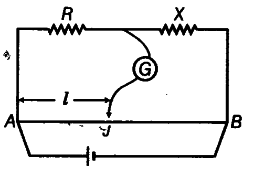# In the meter bridge experiment, the balance point

In the meter bridge experiment, the balance point was observed at J with AJ = l.
(i) The values of R and X are doubled and then interchanged. What would be the new position of balance point?
(ii) If the galvanometer and battery are interchanged at the balance position, how will the balance point get affected?(i) As, per initial balance situation of meter bridge we find that R/X = l/100- l ----(i)
If R and X are doubled and then interchanged. Let new position of null point be at length l’ from end A.
Thus, we have 2X/2R = l’/100 - l’ ----(ii)
Multiplying Eqs. (i) and (ii), we get
1= l/100-l x l’/100-l’
===> l’ = 100-l
(ii) If the galvanometer and the battery are interchanged, there is no effect on the position of balance point at all and balance point remains unchanged.